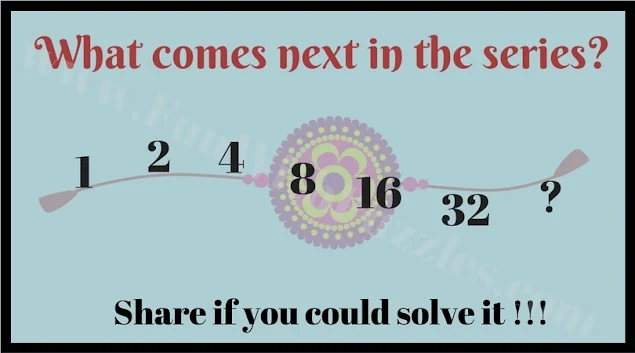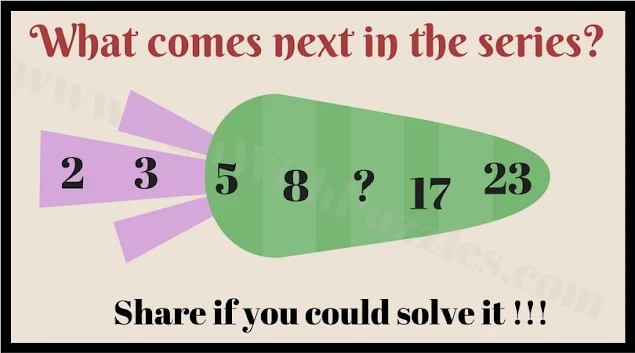The Fun With Puzzle website contains many different types of number puzzles. In some of these number puzzles, one has to find the missing number which will replace the question mark. Some of these numbers of puzzles will require logical reasoning and mathematical skills. Here are number series puzzles in which your challenge is to find the next number in the series. These number puzzles are very easy and designed to be solved by kids. Answers to these number series puzzles are given at the end.1. Can you Find the Next Number in the Series?2. What is the Missing Number in the Series?3. Can you Find the Next Number in the Series?4. What is the Next Number in the Series?5. Can you Find the Next Number in the Series?

1) 64

2) 12

3) 23

4) 4

5) 35